Students can download Maths Chapter 1 Set Language Ex 1.6 Questions and Answers, Notes, Samacheer Kalvi 9th Maths Guide Pdf helps you to revise the complete Tamilnadu State Board New Syllabus, helps students complete homework assignments and to score high marks in board exams.

## Tamilnadu Samacheer Kalvi 9th Maths Solutions Chapter 1 Set Language Ex 1.6

Question 1.
(i) If n(A) = 25, n(B) – 40, n(A∪B) = 50 and n(B’) = 25, find n(A∩B) and n(U).
Solution:
Given, n(A) = 25, n(B) = 40, n(A∪B) = 50 and n(B’) = 25 n(A∩B) = n(A) + n(B) – n(A∪B)
n(A∩B) = n(A) + n(B) – n(A∪B)
n(A∩B) = 25 + 40 – 50
= 65 – 50
= 15
n(U) = n(B) + n(B)’
= 40 + 25
= 65
∴ n(A∩B) = 15 and n(U) = 65

(ii) If n(A) = 300, n(A∪B) = 500, n(A∩B) = 50 and n(B’) = 350, find n(B) and n(U).
Solution:
Given, n(A) = 300, n(A∪B) = 500, n(A∩B) = 50 and n(B’) = 350
n(A∪B) = n(A) + n(B) – n(A∩B)
500 = 300 + n(B) – 50
500 = 250 + n(B)
500 – 250 = n(B)
250 = n(B)
∴ n(B) = 250
n(U) = n(B) + n(B)’
250 + 350 = 600
∴ n(B) = 250 and n(U) = 600Question 2.
If U = {x : x ∈ N, x ≤ 10}, A = { 2, 3, 4, 8, 10} and B = {1, 2, 5, 8, 10}, then verify that n(A∪B) = n(A) + n(B) – n(A∩B)
Solution:
U= {1, 2, 3, 4, 5, 6, 7, 8, 9, 10}
A = {2, 3, 4, 8, 10}; B = {1, 2, 5, 8, 10}
n(U) = 10, n(A) = 5, n(B) = 5
(A∪B) = {2, 3, 4, 8, 10} ∪ {1, 2, 5, 8, 10}
= {1, 2, 3, 4, 5, 8, 10}
∴ n(A∪B) = 7 ……..(1)
(A∩B) = {2, 3, 4, 8, 10} ∩ {1, 2, 5, 8, 10}
= {2, 8, 10}
n(A∩B) = 3
n(A) + n(B) – n(A∩B) = 5 + 5 – 3
= 10 – 3
= 7 ……(2)
From (1) and (2) we get,
n(A∪B) = n(A) + n(B) – n(A∩B)Question 3.
Verify n(A∪B∪C) = n(A) + n(B) + n(C) – n(A∩B) – n(B∩C) – n(A∩C) + n(A∩B∩C) for the following sets.
(i) A = {a, c, e, f, h}, B = {c, d, e, f} and C = {a, b, c, f}
Solution:
A∩B = {a, c, e, f, h} ∩ {c, d, e, f}
= {c, e, f}
B∩C = {c, d, e, f} ∩ {a, b, c, f}
= {c, f}
A∩C = {a, c, e, f, h} ∩ {a, b, c, f}
= {c, f}
(A∩B∩C) = {a, c, e, f, h} ∩ {c, d, e, f} ∩ {a, b, c, f}
= {c, f}
(A∪B∪C) = {a, c, e, f, h} ∪ {c, d, e, f} ∪ {a, b, c, f}
= {a, b, c, d, e, f, h}
n(A∩B) = 3, n(B∩C) = 2, n(A∩C) = 3, n(A∩B∩C) = 2
n(A∪B∪C) = 7……….(1)
n(A) + n(B) + n(C) – n(A∩B) – n(B∩C) – n( A∩C) + n(A∩B∩C)
= 5 + 4 + 4 – 3 – 2 – 3 + 2 = 15 – 8
= 7 ……….(2)
From (1) and (2) we get
n(A∪B∪C) = n(A) + n(B) + n(C) – n(A∩B) – n(B∩C) – n(A∩C) + n(A∩B∩C)

(ii) A= {1, 3, 5}, B = {2, 3, 5, 6}, C = {1, 5, 6, 7}
A∩B = {1, 3, 5} ∩ {2, 3, 5, 6}
= {3, 5}
B∩C = {2, 3, 5, 6} ∩ {1, 5, 6, 7}
= {5, 6}
A∩C = {1, 3, 5} ∩ {1, 5, 6, 7}
= {1, 5}
A∩B∩C = {1, 3, 5} ∩ {2, 3, 5, 6} ∩ {1, 5, 6, 7}
= {5}
A∪B∪C = {1, 3, 5} ∪ {2, 3, 5, 6} ∪ {1, 5, 6, 7}
= {1, 2, 3, 5, 6, 7}
n(A) = 3, n(B) = 4, n(C) = 4
n(A∩B) = 2, n(B∩C) = 2, n(A∩C) = 2
n(A∩B∩C) = 1
n(A∪B∪C) = 6……….(1)
n(A) + n(B) + n(C) – n(A∩B) – n(B∩C) – n(A∩C) + n(A∩B∩C) = 3 + 4 + 4 – 2 – 2 – 2 + 1
= 12 – 6
= 6………(2)
From (1) and (2) we get
n(A∪B∪C) = n(A) + n(B) + n(C) – n(A∩B) – n(B∩C) – n(A∩C) + n(A∩B∩C)Question 4.
In a class, all students take part in either music or drama or both. 25 students take part in music, 30 students take part in drama and 8 students take part in both music and drama. Find
(i) The number of students who take part in only music.
(ii) The number of students who take part in only drama.
(iii) The total number of students in the class.
Solution: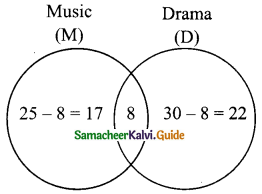Let M be the set of all students take part in music.
Let D be the set of all students take part in drama.
n( M) = 25, n(D) = 30 and n(M∩D) = 8
By using venn-diagram
From the venn – diagram we get.
(i) Number of students take part in only music = 17
(ii) Number of students take part in only drama = 22
(iii) Total number of students in the class = 17 + 8 + 22 = 47

Question 5.
In a party of 45 people, each one likes Tea or Coffee or both. 35 people like tea and 20 people like coffee. Find the number of people who
(i) like both Tea and Coffee.
(ii) do not like Tea.
(iii) do not like Coffee.
Solution: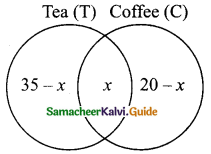Let’T’ be the set of people likes Tea
Let ‘C’ be the set of people likes Coffee
n(T∩C) = 45, n(T) = 35 and n(C) = 20
Let X be the number of people likes both Tea and Coffee.
By using venn diagram
From the venn – diagram we get.
35 – x + x + 20 – x = 45
55 – x = 45
55 – 45 = x
10 = x
(i) People like both tea and coffee = 10
(ii) People do not like tea = 20 – x
= 20 – 10 = 10
(iii) People do not like coffee = 35 – x
= 35 – 10 = 25Question 6.
In an examination 50% of the students passed in mathematics and 70% of students passed in science while 10% students failed in both subjects. 300 students passed in both the subjects. Find the total number of students who appeared in the examination, if they took examination in only two subjects.
Solution:
Let M and S represent the student failed in Mathematics and Science.
Given: Number of students passed in Mathematics is 50%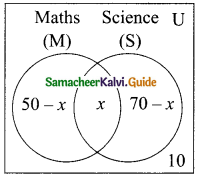∴ Number of students failed in Mathematics = 100 – 50% = 50%
n(M) = 50%
Number of students passed in Science is 70%
∴ Number of students failed in Science = 100 – 70% = 30%
n(S) = 30%
Number of students failed in both the subjects is 10%
n(M∩S) = 10%
n(M∪S)= n(M) + n(S) – n(M∩S)
= 50 + 30 – 10 = 80 – 10 = 70
Given: 70% of the students failed in atleast any one of the subject
∴ 30% of the students passed in atleast any one of the subjects.
30 students passed mean, the total number of students is 100.
∴ 300 students passed means, the total number of students = $$\frac{100 × 300}{30}$$
Total number of students appeared in the examination = 1000

Question 7.
A and B are two sets such that n(A – B) = 32 + x, n(B – A) = 5x and n(A∩B) = x. Illustrate the information by means of a venn diagram. Given that n(A) = n(B). Calculate the value of x.
Solution:
n(A – B) = 32 + x, n(B – A) = 5x
n(A∩B) = x
From the Venn diagram: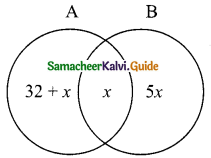Given n( A) = n(B)
32 + x + x = x + 5x
32 + 2x = 6x
32 = 6x – 2x
32 = 4x
x = $$\frac{32}{4}$$ = 8
The value of x = 8Question 8.
Out of 500 car owners investigated, 400 owned car A and 200 owned car B, 50 owned both A and B cars. Is this data correct?
Solution:
Let A be the set of people owned car A
Let B be the set of people owned car B
n( A) = 400, n(B) = 200, n(A∩B) = 50
n(A∪B) = 500………..(1)
n(A) + n(B) – n(A∩B) = 400 + 200 – 50
= 600 – 50
= 550………(2)
From (1) and (2) we get
n(A∪B) ≠ n(A) + n(B) – n(A∩B)
∴ The given data is not correct.

Question 9.
In a colony, 275 families buy Tamil newspaper, 150 families buy English newspaper, 45 families buy Hindi newspaper, 125 families buy Tamil and English newspapers, 17 families buy English and Hindi newspapers, 5 families buy Tamil and Hindi newspapers and 3 families buy all the three newspapers. If each family buy atleast one of these newspapers then find
(i) Number of families buy only one newspaper
(ii) Number of families buy atleast two newspapers
(iii) Total number of families in the colony.
Solution:
Let T, E and H represent families buying Tamil newspaper, English newspaper and Hindi newspaper respectively.
n(T) = 275, n(E) = 150, n(H) = 45
n(T∩E) = 125, n(E∩H) = 17, n(T∩H) = 5
n(T∩E∩H) = 3
Let us represent the given data in Venn diagrams.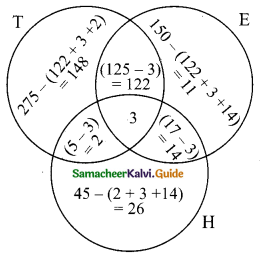(i) Number of families buy only one news paper = 148 + 11 + 26
= 185
(ii) Number of families buy atleast two news paper = 122 + 2 + 14 + 3
= 141
(iii) Total number of families in the colony = 148 + 122 + 11 + 14 + 3 + 2 + 26
= 326Question 10.
A survey of 1000 farmers found that 600 grew paddy, 350 grew ragi, 280 grew corn, 120 grew paddy and ragi, 100 grew ragi and corn, 80 grew paddy and corn. If each farmer grew atleast any one of the above three, then find the number of farmers who grew all the three.
Solution:
Let P, R and C represent sets of farmers grew paddy, ragi and com respectively.
n(P∪R∪C) = 1000, n(P) = 600, n(R) = 350, n(C) = 280
n(P∩R) = 120, n(R∩C) = 100, w(P∩C) = 80 Let the number of farmers who grew all the three be “x”
n(P∪R∪C ) = n(P) + n( R) + n( C) – n(P∩R) – n(R∩C) – n(P∩C) + n(P∩R∩C )
1000 = 600 + 350 + 280 – 120 – 100 – 80 + x = 1230 – 300 + x.
1000 = 930 + x
1000 – 930 = x
70 = x
Number of farmers who grew all the three = 70.

Question 11.
In the adjacent diagram, if n(U) = 125, y is two times of x and z is 10 more than x, then find the value of x, y and z.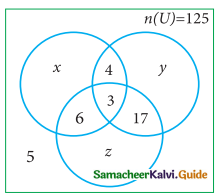Solution:
n(U) = 125
y = 2x and z = x + 10
n(U) = x + 4 + y + 17 + 3 + 6 + z + 5
125 = x + 4 + 2x + 17 + 3 + 6 + x + 10 + 5
125 = 4x + 45
125 – 45 = 4x
80 = 4x
x = 80/4 = 20
y = 2x = 2 × 20 = 40
z = x + 10 = 20 + 10 = 30
∴ The value of x = 20, y = 40 and z = 30.Question 12.
Each student in a class of 35 plays atleast one game among chess, carrom and table tennis. 22 play chess, 21 play carrom, 15 play table tennis, 10 play chess and table tennis, 8 play carrom and table tennis and 6 play all the three games. Find the number of students who play (i) chess and carrom but not table tennis (ii) only chess (iii) only carrom (Hint: Use Venn diagram)
Solution:
Let A, B and C represent students play chess, carrom and table tennis.
n(A) = 22, n(B) = 21 , n(C) = 15
n(A∩C) = 10 , n(B∩C) = 8 , n(A∩B∩C) = 6
Let “x” represent student play chess and carrom but not table tennis.
Let us represent the data in Venn diagram.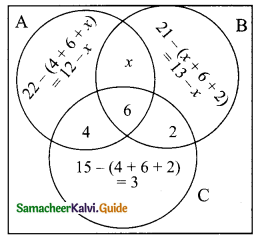From the Venn diagram we get,
Number of students play atleast one game = 35
12 – x + x + 13 – x + 2 + 6 + 4 + 3 = 35
40 – 35 = x
5 = x
(i) Number of students who play chess and carrom but not table tennis = 5
(ii) Number of students who play only chess = 12 – x
= 12 – 5 = 7
(iii) Number of students who play only carrom = 13 – x
= 13 – 5 = 8

Question 13.
In a class of 50 students, each one come to school by bus or by bicycle or on foot. 25 by bus, 20 by bicycle, 30 on foot and 10 students by all the three. Now how many students come to school exactly by two modes of transport?
Solution:
Let B, C and D represent students come to school by bus, bicycle and foot respectively.
n(B∪C∪D) = 50 , n(B) = 25 , n(C) = 20 , n(D) = 30, n(B∩C∩D) = 10
Let x, y and z represent the students come to school exactly by two modes of transport.
Let us represent the given data in Venn diagrams.
<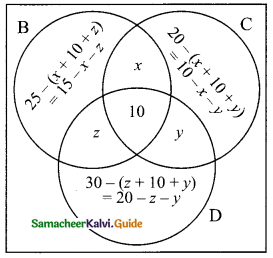Total number of students in the class = 50
15 – x – z + x + 10 – x – y + y + 10 + z + 20 – z – y = 50
55 – x – y – z = 50
55 – 50 = x + y + z
5 = x + y + z
Number of students come to school exactly by two modes of transport = 5